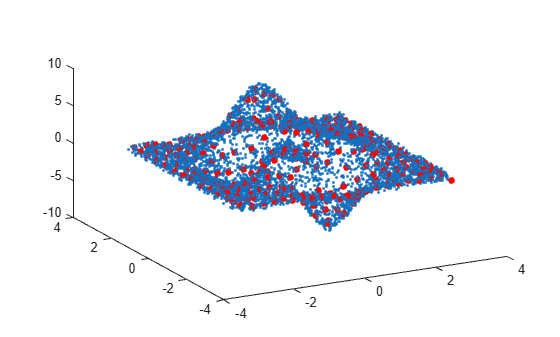Average Similar Data Points Using a Tolerance

This example shows how to use uniquetol to find the average z-coordinate of 3-D points that have similar (within tolerance) x and y coordinates.

Use random points picked from the peaks function in the domain $\left[-3,3\right]×\left[-3,3\right]$ as the data set. Add a small amount of noise to the data.

xy = rand(10000,2)*6-3;
z = peaks(xy(:,1),xy(:,2)) + 0.5-rand(10000,1);
A = [xy z];
plot3(A(:,1), A(:,2), A(:,3), '.')
view(-28,32)Find points that have similar x and y coordinates using uniquetol with these options:

• Specify ByRows as true, since the rows of A contain the point coordinates.

• Specify OutputAllIndices as true to return the indices for all points that are within tolerance of each other.

• Specify DataScale as [1 1 Inf] to use an absolute tolerance for the x and y coordinates, while ignoring the z-coordinate.

DS = [1 1 Inf];
[C,ia] = uniquetol(A, 0.3, 'ByRows', true, ...
'OutputAllIndices', true, 'DataScale', DS);

Average each group of points that are within tolerance (including the z-coordinates), producing a reduced data set that still holds the general shape of the original data.

for k = 1:length(ia)
aveA(k,:) = mean(A(ia{k},:),1);
end

Plot the resulting averaged-out points on top of the original data.

hold on
plot3(aveA(:,1), aveA(:,2), aveA(:,3), '.r', 'MarkerSize', 15)